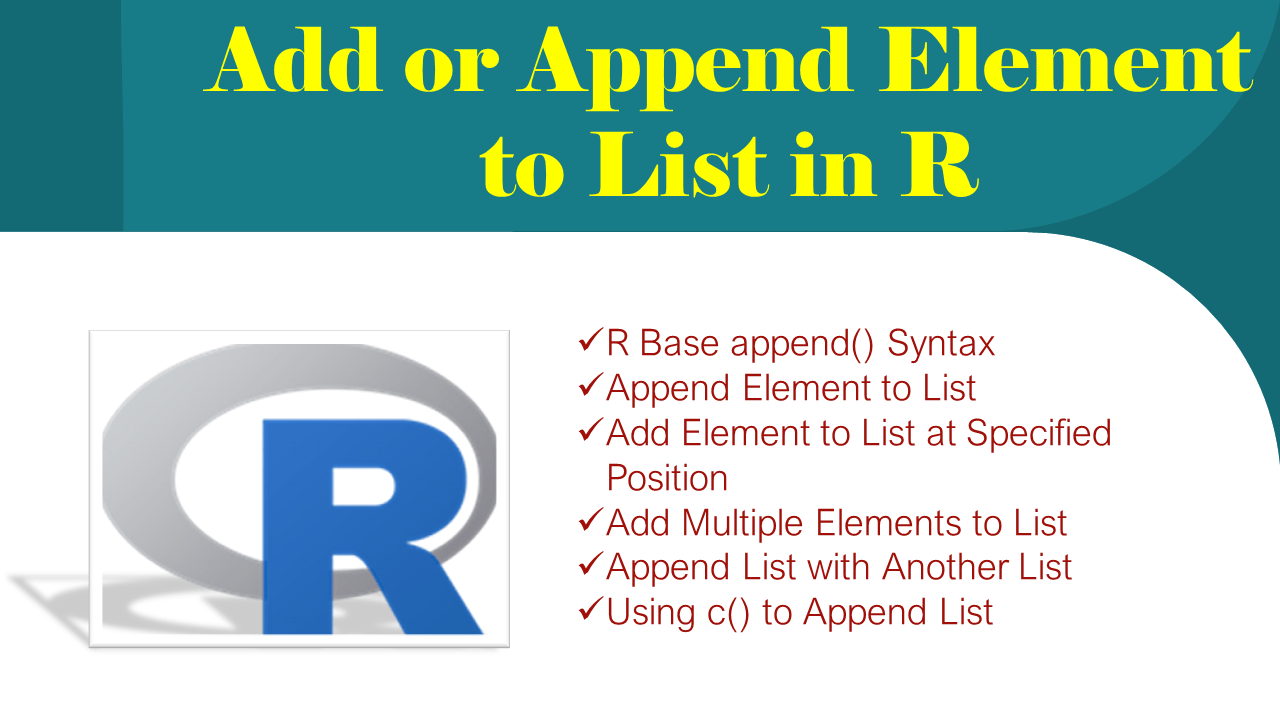# Add or Append Element to List in R?

To add or append an element to the list in R use append() function. This function takes 3 parameters input list, the string or list you wanted to append, and position.

The `list.append()` function from the rlist package can also use to append one list with another in R.

## 1. Quick Examples of Add element to List

Following are quick examples of how to add or append elements to the list in R.

``````
# Quick examples

# Using R base append()
li = list('java','python')
li2 <- append(li,'r')
print(li2)

# Add element to list at specified position
li = list('java','python')
li2 <- append(li,'r',after=1)
print(li2)

# Using list.append()
library('rlist')
li2 <- list.append(li,'r')
print(li2)

# Named List
library('rlist')
li = list(id=11,name='python')
li2 <- list.append(li,pages=34)
print(li2)

# Append list with another list
li = list('java','python')
li2 = list('r','php')
li3 <- append(li,li2)
print(li3)
``````

## 2. R Base append() Syntax

Following is the syntax of the R base append() function

``````
# Syntax of append()
append(x, values, after = length(x))
``````

Parameters

• `x` – Input list
• `values` – string, list or vector.
• `after` – Position to add. Optional. When not specified the element is added at the end of the list.

## 3. Append Element to List

By using the `append()` function let’s add an element to the existing list in R. By default, it adds an element at the end of the list. The following example adds an element `r` to the list.

``````
li = list('java','python')
li2 <- append(li,'r')
print(li2)
``````

Yields below output. Note that we have added item `r` to the list.

``````
# Output
[]
 "java"

[]
 "python"

[]
 "r"
``````

## 4. Add Element to List at Specified Position

Use the `after` parameter to specify the position where you wanted to add the element. The following example adds elements after the first position.

``````
# Add element to list at specified position
li = list('java','python')
li2 <- append(li,'r',after=1)
print(li2)
``````

Yields below output

``````
# Output
[]
 "java"

[]
 "r"

[]
 "python"
``````

## 5. Add Multiple Elements to List

Package `rlist` provides a `list.append()` function to add multiple elements to the list in R. In order to use this function first, you need to install R package by using `install.packages("rlist")` and load it using the `library("rlist")`.

``````
# Add multiple elements to list
library('rlist')
li2 <- list.append(li,'r','50')
print(li2)
``````

Yields below output.

``````
# Output
[]
 "java"

[]
 "python"

[]
 "r"

[]
 "50"
``````

Using this function we can also append elements to the named list.

``````
# Named List
library('rlist')
li = list(id=11,name='python')
li2 <- list.append(li,pages=34)
print(li2)
``````

## 6. Append List with Another List

To append one list with another list pass both lists to the append() function, this adds a second list to the end of the first list and returns a single list.

``````
# Append list with another list
li = list('java','python')
li2 = list('r','php')
li3 <- append(li,li2)
print(li3)
``````

## 7. Using c() to Append List

If you are not aware c() is a combined function that is used to merge the list, vector e.t.c. The following example uses c() to append two lists.

``````
# using c()
li3 = c(li,li2)
print(li3)
``````

## Conclusion

In this article, you have learned how to add or append an element to the list by using R base append(), list.append() from the `rlist` package. You can find the complete example from this article at Github R Programming Examples Project.

## References

### Naveen (NNK)

Naveen (NNK) is a Data Engineer with 20+ years of experience in transforming data into actionable insights. Over the years, He has honed his expertise in designing, implementing, and maintaining data pipelines with frameworks like Apache Spark, PySpark, Pandas, R, Hive and Machine Learning. Naveen journey in the field of data engineering has been a continuous learning, innovation, and a strong commitment to data integrity. In this blog, he shares his experiences with the data as he come across. Follow Naveen @ @ LinkedIn